Next: Multiplication Up: Dyadic Digit Operations Previous: Lowest Terms

# Average

In order to compute the average of two dyadic digits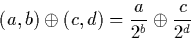it is necessary to compute the value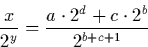with the property that (x,y) is a dyadic digit (ie. x is odd or (x,y) = (0,0)).

We examine the powers of the denominators b and d and come up with three different cases.

Case 1: (b>d)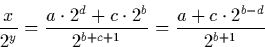As (b > d), we know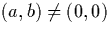, so a is odd,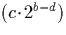is even, and hence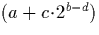is odd. Hence: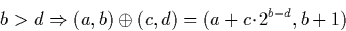Case 2: (d>b)
As average is commutative, we can treat this in exactly the same way as the previous case, but with the arguments reversed. Hence: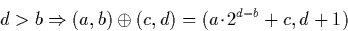Case 3: (b=d)
: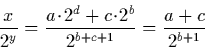However as a and c are odd or zero, (a + c) may be even, or (a+c,b+1) may have a zero first element and non-zero second element. Therefore it is necessary to apply the function described in section A.1 to the pair (a+c,b+1).

Using an almost identical approach, it is possible to define similar functions for finding the average of one digit with the negation of another, addition or subtraction of two digits (with the caveat that if the result of the addition or subtraction is not in the range [-1,1], the pair returned will not be a valid dyadic digit), and other combinations such as addition or subtraction of two dyadic digits (a,b) where a pair of digits (x,y) is returned with the property that: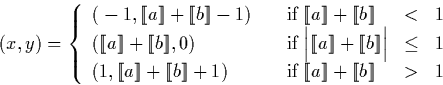Next: Multiplication Up: Dyadic Digit Operations Previous: Lowest Terms
Martin Escardo
5/11/2000